# Graphing Sine and Cosine

Elizabeth Rasmussen, Jeff Calareso
• Author
Elizabeth Rasmussen
• Instructor
Jeff Calareso

Jeff teaches high school English, math and other subjects. He has a master's degree in writing and literature.

Know what sine and cosine waves are and how to graph their respective waves. Read more about the calculation of its period and amplitude through examples here. Updated: 04/26/2022

Show

## Waves

Waves are everywhere. Lights, sounds, energy and more are all made up of waves. A wave is a periodic disturbance that moves energy between two places. Electromagnetic waves are used for cooking food in a microwave. Mechanical waves are found in the waves of the ocean. Sine waves and cosine waves are periodic functions, meaning the functions repeat themselves again and again over a consistent length of values. When referring to sine and cosine waves, the maximum and minimum heights of each wave are constant. Compare sine and cosine to the graph shown of the sound wave. Sound waves can have varying heights for each wave, and they are not necessarily periodic.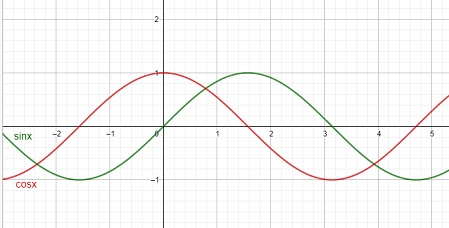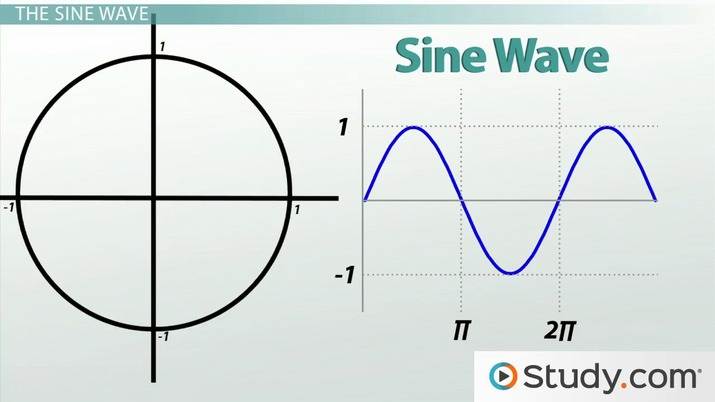An error occurred trying to load this video.

Try refreshing the page, or contact customer support.

Coming up next: Graphing Sine and Cosine Transformations

### You're on a roll. Keep up the good work!

Replay
Your next lesson will play in 10 seconds
• 1:08 The Unit Circle
• 2:01 The Sine Wave
• 4:00 The Cosine Wave
• 5:20 Period
• 6:20 Amplitude
• 7:00 Lesson Summary
Save Save

Want to watch this again later?

Timeline
Autoplay
Autoplay
Speed Speed

## What is a Sine Wave?

The trigonometric function sine is defined as {eq}y=sin(x) {/eq}. The function oscillates around the line y = 0. This means the function goes up and down around the x-axis repeatedly. A sine wave, also called a sinusoidal wave, is represented by the sine function and is a smooth curve that repeats itself periodically.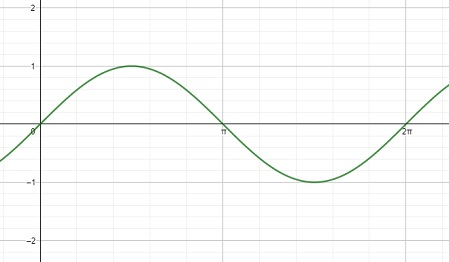Sine waves are used to model musical tones, ocean tides, how far away one planet is from another, and stockbrokers even use sine waves to predict the best time to sell or buy a stock.

The reason sine waves garner such important status in trigonometry is that so many real-life applications (as mentioned in the previous paragraph) can be modeled with sinusoidal waves but not with any other trigonometric wave. And while these sine waves may not always match the situation perfectly, they do a much better job approximating the wave than most other functions ever could.

Listed below is some common terminology when talking about sine waves.

• Amplitude: The height of the function from the line y = 0 to its maximum y-value or minimum y-value
• Period: How long it takes the function to repeat itself; the smallest value of p such that {eq}f(x)=f(x+p) {/eq} for all x.
• Phase shift: The number of units the function shifts left or right
• Frequency: How many times the wave repeats itself in an interval of length {eq}2\pi {/eq}

### Sine Function

A sine wave is represented by the following sine function: {eq}y=asin(bx-c) {/eq} where

• |a| is the amplitude
• x is the variable
• {eq}\dfrac{2\pi}{|b|} {/eq} is the period
• {eq}\dfrac{c}{b} {/eq} is the phase shift

The basic sine function is {eq}y=sin(x) {/eq} where a = 1, b = 1, c = 0 and d = 0. Thus, the amplitude of this function is 1, the period is {eq}\dfrac{2\pi}{1} = 2\pi {/eq}, and the phase shift is {eq}\dfrac{0}{1}=0 {/eq}.

Five important points on the graph of {eq}sin(x) {/eq} are {eq}(0,0), (\dfrac{\pi}{2}, 1), (\pi, 0), (\dfrac{3\pi}{2}, -1), (2\pi, 0) {/eq}

These points are the locations of the maximum y-value, the minimum y-value, and the three points where the function intersects the x-axis.

### Graphing Sine Waves

Careful attention to order is necessary to graph a sine wave correctly.

• Step 1: Identify the amplitude, |a|.
• Step 2: Find the period by calculating {eq}\dfrac{2\pi}{|b|} {/eq}.
• Step 3: Identify the phase shift, {eq}\dfrac{c}{b} {/eq}.
• Step 4: Find the new x-values and y-values of the five main points.
• Step 5: Plot the new points.
• Step 6: Connect all the points with a smooth continuous line.

#### Example: Graph the function {eq}y=3sin(2x-pi) {/eq}.

Step 1: Identify the amplitude, {eq}|a| {/eq}.

{eq}|a|=|3|=3 {/eq}, so the amplitude is 3. This means the maximum y-value is 3 and the minimum y-value is -3.

Step 2: The period is found with the formula {eq}\dfrac{2\pi}{|b|} {/eq}.

{eq}\dfrac{2\pi}{|2|} = \pi {/eq}. The function will repeat itself after {eq}\pi {/eq} units.

Step 3: The phase shift is {eq}\dfrac{c}{|b|} = \dfrac{\pi}{2} {/eq}. The graph of the function shifts to the right by {eq}\dfrac{\pi}{2} {/eq} units.

Step 4: Find the new x and y-values.

In the original {eq}y=sin(x) {/eq} graph, the five important x-values were {eq}0, \dfrac{\pi}{2}, \pi, \dfrac{3\pi}{2}, 2\pi. {/eq}

To find the x-values of this function after the phase shift and shrinking of the period, we need to solve the following equations where the argument of the sine function is set equal to the original x-values:

{eq}2x-\pi=0\\ 2x-\pi= \dfrac{\pi}{2}\\ 2x-\pi= \pi\\ 2x-\pi= \dfrac{3\pi}{2}\\\ 2x-\pi= 2\pi {/eq}

First, add {eq}\pi {/eq} to each side.

{eq}2x=\pi\\ 2x= \dfrac{3\pi}{2}\\ 2x= 2\pi\\ 2x= \dfrac{5\pi}{2}\\ 2x= 3\pi {/eq}

Then, divide each side of the equations by 2.

{eq}x=\dfrac{\pi}{2}\\ x= \dfrac{3\pi}{4}\\ x= \pi\\ x= \dfrac{5\pi}{4}\\ x= \dfrac{3\pi}{2} {/eq}

These five values are the new locations of the minimum y-value, maximum y-value, and the x-intercepts.

Finding the y-values is simpler: multiply every y value by the amplitude. The original y-values were 0, 1, 0, -1, 0 and, once multiplied by the amplitude of 3, become 0, 3, 0, -3, 0.

Thus the points on the graph are

{eq}(\dfrac{\pi}{2},0)\\ (\dfrac{3\pi}{4},3)\\ (\pi,0)\\ (\dfrac{5\pi}{4},-3)\\ (\dfrac{3\pi}{2},0) {/eq}

Step 5: Plot the new x-values and y-values.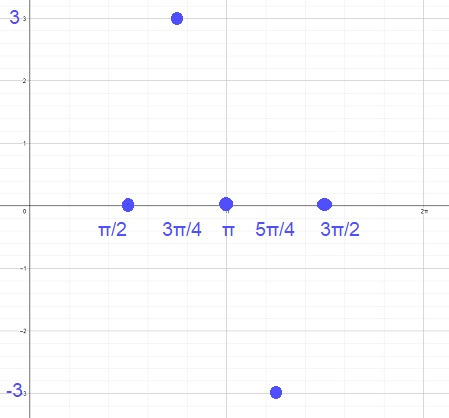Step 6: Connect all the points with a smooth continuous line.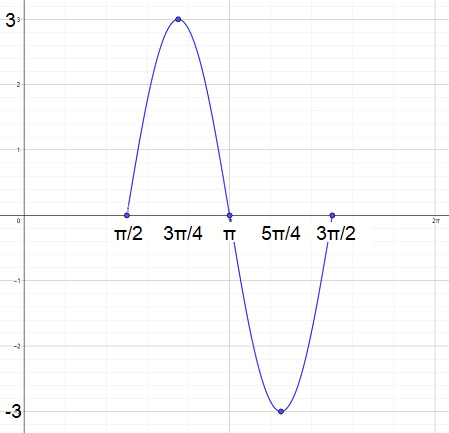That is the completed sine graph.

## What is a Cosine Wave?

The cosine wave is the same as the sine wave but shifted {eq}\dfrac{\pi}{2} {/eq} units to the right. That is, {eq}sin(x)=cos(x-\dfrac{\pi}{2}) {/eq}. Because of this equality, everything that holds true for the sine wave holds true for the cosine wave. Since cosine is shifted to the right by {eq}\pi/2 {/eq} units, there is a new set of five points: {eq}(0, 1), (\dfrac{\pi}{2}, 0), (\pi, -1), (\dfrac{3\pi}{2}, 0), (2\pi, 1) {/eq}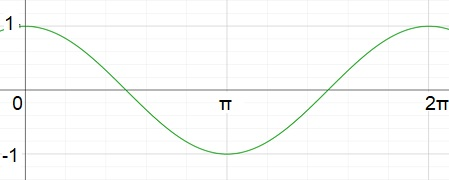### Cosine Function

Recall the definitions from the sine wave function:

• Amplitude: the height of the function from the line y = 0 to its maximum y-value or minimum y-value.
• Period: How long it takes the function to repeat itself. The smallest value of p such that {eq}f(x)=f(x+p) {/eq} for all x.
• Phase shift: The number of units the function is shifted left or right.

A Cosine wave is represented by the following cosine function: {eq}y=acos(bx-c) {/eq} where

• |a| is the amplitude
• x is the variable
• {eq}\dfrac{2\pi}{|b|} {/eq} is the period
• {eq}\dfrac{c}{b} {/eq} is the phase shift

This is the graph of the basic cos(x) function where a = 1, b = 1, and c = 0.### Graphing Cosine Waves

The steps for graphing the cosine function are the same as those for graphing the sine function.

• Step 1: Identify the amplitude, |a|.
• Step 2: Find the period by calculating {eq}\dfrac{2\pi}{|b|} {/eq}.
• Step 3: Identify the phase shift, {eq}\dfrac{c}{b} {/eq}.
• Step 4: Find the new x-values and y-values.
• Step 5: Plot the new x-values and y-values.
• Step 6: Connect all the points with a smooth continuous line.

#### Example: Graph the function {eq}y=5cos(\dfrac{\pi}{3} x-\dfrac{\pi}{2}) {/eq}.

Step 1: Identify the amplitude, |a|.

a = |5| = 5.

Step 2: Find the period by calculating {eq}\dfrac{2\pi}{|b|} {/eq}.

{eq}\dfrac{2\pi}{\dfrac{\pi}{3}}={2\pi}*\dfrac{3}{\pi}=6 {/eq}.

Step 3: Identify the phase shift, {eq}\dfrac{c}{b} {/eq}.

To unlock this lesson you must be a Study.com Member.

#### What is sine wave used for?

Sine waves are used to model waves occurring in the real world. This can range from uses in the stock market, to modeling the ocean tides, to examining shocks from earthquakes.

#### How do you graph a sine wave?

Identify the amplitude, period, phase shift, and the 5 main points. Carefully plot the points on a graph and then connect them with a smooth continuous curve.

#### What is an example of a cosine function?

An example of a cosine function is cos(x). Another example is 5cos(x). This vertically stretches the function cos(x) by 5 units. In the function cos(2x), the function is shrunk horizontally by a factor of 2. Finally, cos(x-3) shifts the graph of cos(x) 3 units to the right.

#### What are the characteristics of a sine wave?

The sine function is an S-shaped curve that repeats itself every period of 2pi. The maximum y-value is 1 and the minimum y-value is -1.

#### How do sine waves differ from cosine waves?

Sine waves are different than cosine waves in that the sine wave is a quarter cycle behind the cosine wave. If one were to shift the sine wave by pi/2 to the left, it is identical in every respect to the cosine wave.

### Register to view this lesson

Are you a student or a teacher?